Consider allowing JavaScript. Otherwise, you have to be proficient in readingsince formulas will not be rendered. Furthermore, the table of contents in the left column for navigation will not be available and code-folding not supported. Sorry for the inconvenience.

Examples in this article were generated with4.2.0 by the packages JuliaCall,1 Rmpfr,2 microbenchmark,3 and rational,4 spiced with a little Python5 and Julia6 run on a Core i5-8265U @ 1.60GHz (1/4 cores) on Windows 11 build 22000.

• The right-hand badges give the respective section’s ‘level’.

1. Basics – requiring no or only limited expertise in computer languages or spreadsheets.

1. These sections are the most important ones. They are – hopefully – easily comprehensible even for novices.

1. A somewhat higher knowledge of computer languages (and especially R) is required. May be skipped or reserved for a later reading.

1. An advanced knowledge of R is required. Definitely not recommended for beginners.

1. If you are not a neRd or computer geek, skipping is recommended. Suggested for experts but might be confusing for others.
• Click to show / hide R code.

# Introduction

Shall we bother about numeric precision?

It all started with an observation in Excel.

When you enter …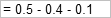you will getWell, as expected. But when you enter …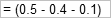you will get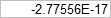What? Strange at least.

top of section ↩︎

# Other Software

OpenOffice CalcBoth without and with parentheses. Amazing!

In all others below it doesn’t matter whether parentheses are used or not.

Gnumeric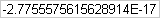MaximaGNU Octave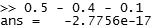Python

x = [0.5, -0.4, -0.1]
print(sum(x))
# -2.7755575615628914e-17

Julia

x = [0.5, -0.4, -0.1]
# 3-element Vector{Float64}:
#   0.5
#  -0.4
#  -0.1
print(sum(x))
# -2.7755575615628914e-17

What about R?

0.5 - 0.4 - 0.1
#  -2.775558e-17

Gimme more digits, pleeze!

print(format(0.5 - 0.4 - 0.1, digits = 15))
#  "-2.77555756156289e-17"

Root cause analysis:

m <- matrix(data = c(0.5, -0.4, -0.1,
(0.5 - 0.4 - 0.1)),
dimnames = list(c("a", "b", "c",
"sum(a, b, c)"),
"value"))
print(m, digits = 17)
#                                value
# a             5.0000000000000000e-01
# b            -4.0000000000000002e-01
# c            -1.0000000000000001e-01
# sum(a, b, c) -2.7755575615628914e-17

Note the ultimate decimal place of b and c!

# Julia
b = -0.4;
typeof(b)
# Float64
x = prevfloat(b)
# -0.4000000000000001
y = nextfloat(b)
# -0.39999999999999997
println(b - x); print(y - a)
# 5.551115123125783e-17
# -5.4
BigFloat(b)
# -0.40000000000000002220446049250313080847263336181640625
big"-0.4"
# -0.4000000000000000000000000000000000000000000000000000000000000000000000000000009

Sooner or later we reach the numeric resolution.

# Julia
b = -0.4;
x = BigFloat(-0.4);
y = big"-0.4";
println(typeof(b)); println(typeof(x)); print(typeof(y))
# Float64
# BigFloat
# BigFloat
println(x - b); print(y - b)
# 0.0
# 2.220446049250313080847263336181640624999999999999999999999999913638314449055554e-17
x = [BigFloat(0.5), BigFloat(-0.4), BigFloat(-0.1)]
# 3-element Vector{BigFloat}:
#   0.5
#  -0.40000000000000002220446049250313080847263336181640625
#  -0.1000000000000000055511151231257827021181583404541015625
sum(x)
# -2.77555756156289135105907917022705078125e-17
x = [big"0.5", big"-0.4", big"-0.1"]
# 3-element Vector{BigFloat}:
#   0.5
#  -0.4000000000000000000000000000000000000000000000000000000000000000000000000000009
#  -0.1000000000000000000000000000000000000000000000000000000000000000000000000000002
sum(x)
# -1.079521069386805578173293982850049946389500045554535173127962933771073975395303e-78

However, the ‘higher precision’ is a delusion.

Reach for the stars, even if you have to stand on a cactus.
Susan Longacre

Arbitrarily accurate computation with R is provided by the package Rmpfr.

library(Rmpfr)
x <- mpfr(c(0.5, -0.4, -0.1), prec = 260)
x
sum(x)
# 3 'mpfr' numbers of precision  260   bits
#                                                         0.5
#    -0.40000000000000002220446049250313080847263336181640625
#  -0.1000000000000000055511151231257827021181583404541015625
# 1 'mpfr' number of precision  260   bits
#  -2.77555756156289135105907917022705078125e-17

OK, more digits but still not what we expect.

Another example:

x <- seq(0.40, 0.43, 0.01)
x
print(x, digits = 17)
mpfr(x, prec = 260)
#  0.40 0.41 0.42 0.43
#  0.40000000000000002 0.41000000000000003
#  0.42000000000000004 0.42999999999999999
# 4 'mpfr' numbers of precision  260   bits
#   0.40000000000000002220446049250313080847263336181640625
#   0.41000000000000003108624468950438313186168670654296875
#   0.42000000000000003996802888650563545525074005126953125
#  0.429999999999999993338661852249060757458209991455078125

Actually it turned out to be the most frequently asked question about R, the (in)famous FAQ 7.31.7

What happened here? We have fallen into the trap of floating point arithmetic.

# History

The first attempts to build something we call now a computer8 were made by Charles Babbage prior to 1840. Of course, both the Difference Engine and the Analytical Engine were – as their names suggest – purely mechanical. The latter was already programmable (its instruction set developed by Ada Lovelace). Their numeral system was decimal and hence, the examples above likely would have easily worked.

In 1941 Konrad Zuse completed construction of the Z3, which was the first digital computer. Once you deal with electrics (relays, vacuum tubes), it’s clear why Zuse decided to work with binary digits.
The signal is either off or on .

# Numeral Systems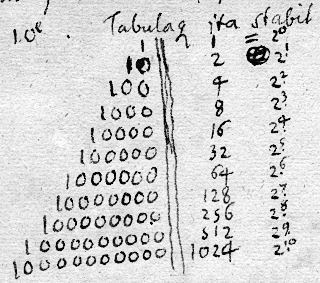Fig.1 Binary numeral system – Gottfried Wilhelm Leibniz 1697.
De Dyadicis Signature LH XXXV, III B 1, Bl. 1-4

To convert a decimal number to binary digit, we have to split the number into its integer and fractional part. Procedure for 10.125 as an example.

10 / 2 = 5: r 0
5 / 2 = 2: r 1
2 / 2 = 1: r 0
1 / 2 = 0: r 1

reordered from least significant bit upwards → 2

0.125 × 2 = 0.25: d 0
0.25  × 2 = 0.50: d 0
0.50  × 2 = 1   : d 1

complete: [10.125]10 = [1010.001]2

Now we will see why 0.5–0.4–0.1 is so difficult in the binary system.

[0.5]10 = [0.1]2
[0.4]10 = [0.011001100110011001100110011001100110…]2 = [0.0110011]2
[0.1]10 = [0.0001100110011001100110011001100110011…]2 = [0.00011]2

Only real numbers $$\small{\mathbb{R}\subset2^{\,\mathbb{Z}}}$$, where $$\small{\mathbb{Z}}$$ is an integer {…, –1, 0, 1, …}, can be converted to a binary number without a remainder. This works for 0.5 (= 2–1) but not for 0.4 and 0.1; we get periodic binary numbers, which cannot be stored in the binary format without truncation.9 10

According to IEEE 754 a binary in double precision holds 64 bits (where 1 bit is the sign, 11 the exponent, and 52 the mantissa). That translates into ~15.7 digits decimal.

log(2^52, 10)
abs(log(.Machine$double.eps, 10)) #  15.65356 #  15.65356 Then Ohlbe posted this example, which cought me on the wrong foot first. It boiled down to the question why the second one works (although 5 is not a multiple of two): 0.5 - 0.4 - 0.1 == 0 5 - 4 - 1 == 0 #  FALSE #  TRUE 10 = 2 10 = 2 10 = 2 Easy. I overlooked that the conversion to a binary works for any integer $$\small{\mathbb{Z}}$$ within the range of $$\small{\left\{-2^{-31}=-2,147,483,648\,\ldots\,2^{31}-1=2,147,483,647\right\}}$$. -10 = -2 10 = +2 a <- .Machine$integer.max
print(a)
print(class(a))
#  2147483647
#  "integer"
b <- as.integer(1)
c <- a + b
# Warning in a + b: NAs produced by integer overflow
print(c)
class(c)
#  NA
#  "integer"

Does not work because the integer range is exhausted.

d <- as.numeric(1) # double precision (float)
e <- a + d
print(e)
class(e)
#  2147483648
#  "numeric"

We get what we expect because a type conversion is performed.

a <-  0.5
b <- -0.4
c <- -0.1
cat("\n", a + b + c == (a +  b) + c,
"\n", a + b + c ==  a + (b  + c),
"\n", a + b + c == (a +  b  + c), "\n")
a <-  5
b <- -4
c <- -1
cat("\n", a + b + c == (a +  b) + c,
"\n", a + b + c ==  a + (b  + c),
"\n", a + b + c == (a +  b  + c))
#
#  TRUE
#  FALSE
#  TRUE
#
#  TRUE
#  TRUE
#  TRUE
# Python
a =  0.5
b = -0.4
c = -0.1
print("\n", a + b + c == (a +  b) + c,
"\n", a + b + c ==  a + (b  + c),
"\n", a + b + c == (a +  b  + c))
a =  5
b = -4
c = -1
print("\n", a + b + c == (a +  b) + c,
"\n", a + b + c ==  a + (b  + c),
"\n", a + b + c == (a +  b  + c))
#
#  True
#  False
#  True
#
#  True
#  True
#  True
# Julia
a =  0.5;
b = -0.4;
c = -0.1;
print("\n", a + b + c == (a +  b) + c,
"\n", a + b + c ==  a + (b  + c),
"\n", a + b + c == (a +  b  + c), "\n")
#
# true
# false
# true
a =  5;
b = -4;
c = -1;
print("\n", a + b + c == (a +  b) + c,
"\n", a + b + c ==  a + (b  + c),
"\n", a + b + c == (a +  b  + c))
#
# true
# true
# true

When dealing with floating point arithmetic, the order (and parentheses) matter (THX to mittyri). No problems with integers.

# To Do and Not to Do

If you want to compare double precision numbers, say, in the logical construct of a script, i.e., if(), while(), repeat(), do not use these goodies:

a <- 0.5 - 0.4 - 0.1
b <- 0
a == b          # most commonly used
identical(a, b) # not better
#  FALSE
#  FALSE

BTW, identical() can give unexpected results.

a <- 2147483647
b <- 2147483647L
class(a)
class(b)
a == b
identical(a, b)
#  "numeric"
#  "integer"
#  TRUE
#  FALSE

Here testing for equality passes because the numbers are not above to maximum possible integer of the system, i.e., 231–1 (in R that’s .Machine$integer.max). However, testing with identical()fails because it compares not only the values but also their classes. Instead use: a <- 0.5 - 0.4 - 0.1 b <- 0 all.equal(a, b) a <- 2147483647 b <- 2147483647L all.equal(a, b) #  TRUE #  TRUE Only this function compares numbers – irrespective of their classes – based on the numeric resolution of a 64 bit double precision numeric, which is $$\small{\approx2.220446\cdot10^{-16}}$$. Actually the comparison is performed at its square root or $$\small{\approx1.49011610^{-8}}$$. The source of all.equal() is lengthy but we can mimick what goes on behind the curtain. a <- 2147483647 b <- 2147483647L all.equal(a, b) sqrt(.Machine$double.eps) >= abs(a - b)
#  TRUE
#  TRUE

We can get $$\small{\pi}$$ with up to 16 correct significant digits.

cat(formatC(pi, digits = 16, small.mark = "\u2219",
small.interval = 3), "\n")
# 3.141·592·653·589·793

However, that was a lucky punch because as we have seen above, the 16th is already inaccurate. But again, don’t dare to ask for more digits. Anything beyond the 15th significant digit is just ‘noise’.

sm <- "\u2219"
cat("64 bit max     =", formatC(pi,
digits = 15,
small.mark = sm,
small.interval = 3),
"\n64 bit \u2018noise\u2019 =", formatC(pi,
digits = 31,
small.mark = sm,
small.interval = 3),
"\ncorrect        =", paste0("3.141", sm, "592", sm, "653", sm,
"589", sm, "793", sm, "238", sm,
"462", sm, "643", sm, "383", sm,
"279 \u2026"), "\n")
# 64 bit max     = 3.141·592·653·589·79
# 64 bit ‘noise’ = 3.141·592·653·589·793·115·997·963·468·544
# correct        = 3.141·592·653·589·793·238·462·643·383·279 …

Honestly, I don’t know why it is possible to ask R for more than 15 digits. At least it should issue a message like
Please use your wetware before asking!

We remember from trigonometry that $$\small{\sin\pi=0}$$. Given the above, can we really hope for that?

sin(pi)
#  1.224606e-16

Now we shouldn’t be surprised any more.

# Python
import math
print(math.sin(math.pi))
# 1.2246467991473532e-16
# Julia
sin(pi)
# 1.2246467991473532e-16
sin(BigFloat(pi))
# 1.096917440979352076742130626395698021050758236508687951179005716992142688513354e-77

Closer to zero. Will we fare better with Rmpfr?

pi. <- Const("pi", prec = 260)
sin(pi.)
# 1 'mpfr' number of precision  260   bits
#  1.7396371592546498568836643545648074661258190954152778051042783221068713118047497e-79

Seems that to hope for zero is futile. However, this ‘better’ result comes with a price, speed.

library(microbenchmark)
res <- microbenchmark(sin(pi), sin(pi.), times = 1000L)
options(microbenchmark.unit = "relative")
print(res, signif = 4)
# Unit: relative
#      expr min    lq  mean median    uq max neval cld
#   sin(pi) NaN   1.0   1.0    1.0   1.0   1  1000  a
#  sin(pi.) Inf 336.9 333.8  336.7 338.7 178  1000   b

A funky one11 discovered by mittyri: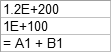Not only in Excel…

1.2e+200 + 1e+100
#  1.2e+200
# Python
print(1.2e+200 + 1e+100)
# 1.2e+200
# Julia
1.2e+200 + 1e+100
# 1.2e200
BigFloat(1.2e+200) + BigFloat(1e+100)
# 1.200000000000000031665409735558622623636694369262012649966820080464248350755499e+200

Bad luck (exhausting the double precision). It doesn’t make sense to hope for the correct
12 000 000 000 000 000 000 000 000 000 000 000 000 000 000 000 000 000 000 000 000 000 000 000 000 000 000 000 000 000 000 000 000 001 000 000 000 000 000 000 000 000 000 000 000 000 000 000 000 000 000 000 000 000 000 000 000 000 000 000 000 000 000 000 000 000 000.
Can you spot the 1?

We learned that we shouldn’t divide by zero. That’s one of the most annoying errors when you start writing own code.

Developers of spreadheets didn’t want to confuse users who never made it beyond basic maths.An even very small number works but zero doesn’t. But is that correct? Ever came across $$\small{\lim\,f(x)}$$?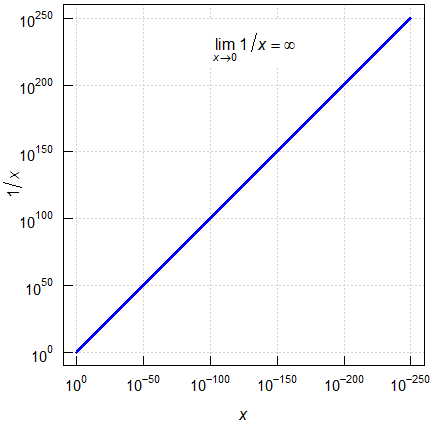Fig. 3 Dividing by small numbers in R.

df             <- data.frame(x = c(0, 1, 1e-10, 1e-250),
y = 1 / c(0, 1, 1e-10, 1e-250))
df$z <- 1 / df$y
for (i in 1:nrow(df)) {
df$comp[i] <- isTRUE(all.equal(df$x[i], df$z[i])) } names(df)[2:4] <- c("y = 1/x", "z = 1/y", "z == x") print(df, row.names = FALSE) # x y = 1/x z = 1/y z == x # 0e+00 Inf 0e+00 TRUE # 1e+00 1e+00 1e+00 TRUE # 1e-10 1e+10 1e-10 TRUE # 1e-250 1e+250 1e-250 TRUE Not only $$\small{1/0=\infty}$$ but also $$\small{1/\infty=0}$$. Nice, though that’s not helpful. summary(df[, 1:3]) # x y = 1/x z = 1/y # Min. :0.00 Min. : 1.0e+00 Min. :0.00 # 1st Qu.:0.00 1st Qu.: 7.5e+09 1st Qu.:0.00 # Median :0.00 Median :5.0e+249 Median :0.00 # Mean :0.25 Mean : Inf Mean :0.25 # 3rd Qu.:0.25 3rd Qu.: Inf 3rd Qu.:0.25 # Max. :1.00 Max. : Inf Max. :1.00 # Julia x = [0, 1, 1e-10, 1e-250]; y = 1 ./x; z = 1 ./y; a = [x y z] # 4×3 Matrix{Float64}: # 0.0 Inf 0.0 # 1.0 1.0 1.0 # 1.0e-10 1.0e10 1.0e-10 # 1.0e-250 1.0e250 1.0e-250 a[:, 3] == a[:, 1] # true What about another infamous candidate, namely $$\small{\log_{e}0}$$?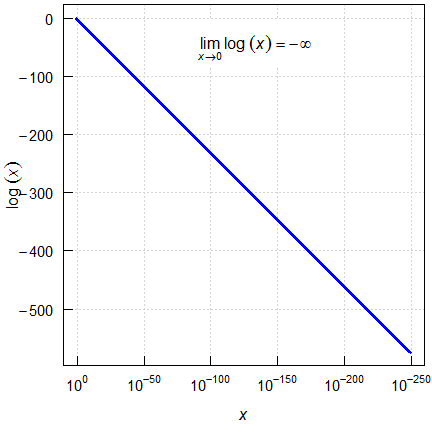Fig. 4 $$\small{\log_{e}x}$$ in R. df <- data.frame(x = c(exp(1), exp(1) / 1e10, exp(1) / 1e250, 0), y = c(log(exp(1)), log(exp(1) / 1e10), log(exp(1) / 1e250), log(0))) df$z           <- exp(df[, 2])
for (i in 1:nrow(df)) {
df$comp[i] <- isTRUE(all.equal(df$x[i], df$z[i])) } names(df)[2:4] <- c("y = log(x)", "z = exp(y)", "z == x") print(df, row.names = FALSE) # x y = log(x) z = exp(y) z == x # 2.718282e+00 1.00000 2.718282e+00 TRUE # 2.718282e-10 -22.02585 2.718282e-10 TRUE # 2.718282e-250 -574.64627 2.718282e-250 TRUE # 0.000000e+00 -Inf 0.000000e+00 TRUE Similarly to the reciprocal of zero above, no fear of infinity: $$\small{\log_{e}0=-\infty}$$ and $$\small{\exp(-\infty)=0}$$. # Julia x = [exp(1), exp(1)/1e10, exp(1)/1e250, 0]; y = log.(x); z = exp.(y); a = [x y z] # 4×3 Matrix{Float64}: # 2.71828 1.0 2.71828 # 2.71828e-10 -22.0259 2.71828e-10 # 2.71828e-250 -574.646 2.71828e-250 # 0.0 -Inf 0.0 a[:, 3] == a[:, 1] # false a[[2, 3], 3] == a[[2, 3], 1] # false a[[1, 4], 3] == a[[1, 4], 1] # true Interesting. Contrary to R, the second and third row fail, where the first and fourth pass. Yep, the last had $$\small{-\infty}$$ as an intermediate result and no problems with $$\small{\exp(-\infty)}$$. For simplicity we can say that $$\small{\log_{e}0}$$ is undefined. It would not be a good idea to trust in a mathematically correct value which distorts subsequent calculations. It is reasonable to assume that concentrations $$(\small{x \in \mathbb{R}^+)}$$ follow a lognormal distribution. The geometric mean should not work if a value is zero because it is outside the domain of the lognormal distribution. Say, we have an arbitrary long vector of identical values and add a single zero-element to the vector. numbers <- 999 value <- 1L x <- c(rep(value, numbers), 0L) gm <- exp(mean(log(x), na.rm = TRUE)) cat(paste0(numbers, " identical values (", value, "), one zero.\n")) summary(x) cat("geometric mean:", gm, "\n") cat("x is", typeof(x), "\ngeometric mean is", typeof(gm)) # 999 identical values (1), one zero. # Min. 1st Qu. Median Mean 3rd Qu. Max. # 0.000 1.000 1.000 0.999 1.000 1.000 # geometric mean: 0 # x is integer # geometric mean is double import StatsBase x = repeat(, 999); x = push!(x, 0); gm = StatsBase.geomean(x); StatsBase.describe(x) # Summary Stats: # Length: 1000 # Missing Count: 0 # Mean: 0.999000 # Minimum: 0.000000 # 1st Quartile: 1.000000 # Median: 1.000000 # 3rd Quartile: 1.000000 # Maximum: 1.000000 # Type: Int64 print("geometric mean: ", gm, "\n", "Type is ", typeof(gm)) # geometric mean: 0.0 # Type is Float64 Because: $\small{\begin{array}{l} x_{i=1\ldots n-1}=1,\;x_{i=n}=0\\ x=\left\{1,\ldots,1,0\right\}\\ \log_{e}x=\left\{0,\ldots,0,-\infty\right\}\\ \overline{\log_{e}x}=\sum \left\{0,\ldots,0,-\infty\right\}/n=-\infty\\ \overline{x}_\textrm{geom.}=\exp(-\infty)=0\;\tiny{\square} \end{array}}$ Note also the type conversion in R and Julia. Though x consists of integers, the geometric mean is a double precision float. # For Geeks only! # EpilogueFig. 4 Free42 Decimal v3.0.10 That’s fascinating. When I copied the output of π to the clipboard it showed 34 (‼) significant digits 3.141592653589793238462643383279503, which is correct to the penultimate digit; the last one is rounded up from 28. How is that possible? Numbers are represented with a 34 digit mantissa and an exponent from 10−6,413 to 106,144, i.e., are handled in quadruple precision (128 bit)!13 Even the original HP-42S (1988!) represented numbers with a 12 digit mantissa and an exponent from 10−499 to 10499, which is larger than the IEEE-754 double precision range of 10−308 to 10308. I’m impressed. What about M$?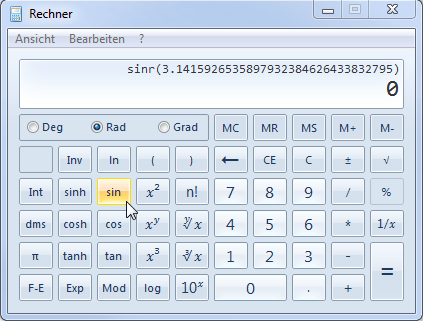Fig. 5 Windows 7 calc.exe

gives 32 correct significant digits. How?
zero? Sorry, that’s beyond me.

# Post Scriptum

Just to set all of this into perspective:
The diameter of human hair is ≈10–12 of the the earth-moon distance. One Nanometer is ≈10–13 of the earth’s equator. Should we really be concerned about an ‘error’ which is three or more orders of magnitude smaller? 😉
Our measurements are likely never that precise anyway. Furthermore, the standard defines sophisticiated rounding routines. Hence, in repeated calculations the error will not propagate upwards.

Hence, the answer to the question
Shall we bother about numeric precision?
is in general
No.

The physical constant with the highest precision is the Faraday constant F (9.648 533 212 331 001 84·104 A·s·mol–1) with 18 significant digits. Unless you are an experimental physicist, double precision numbers are fine. Otherwise, opt for a language supporting extended precision like GCC C/C++, Clang, Intel C++, Object Pascal, Racket, Swift, or get a suitable scientific pocket calculator.

Acknowledgment

Members of the BEBA-Forum: ElMaestro, mittyri, Ohlbe, PharmCat, Shuanghe, and zizou.Helmut Schütz 2022
R and all packages GPL 3.0, rational, Free42, and pandoc GPL 2.0, Python Open Source (GPL compatible), Julia MIT.
1st version March 14, 2021. Rendered June 18, 2022 20:39 CEST by rmarkdown via pandoc in 1.85 seconds.

Footnotes and References

1. Li C, Lai R, Grominski D, Teramo N. JuliaCall: Seamless Integration Between R and ‘Julia’. Package version 0.17.4. 2021-05-14. CRAN.↩︎

2. Maechler M, Heiberger RM, Nash JC, Borchers HW. Rmpfr: R MPFR - Multiple Precision Floating-Point Reliable. Package version 0.8.9. 2022-06-02. CRAN.↩︎

3. Mersmann O, Beleites C, Hurling R, Friedman A, Ulrich JM. microbenchmark: Accurate Timing Functions. Package version 1.4.9. 2021-11-07. CRAN.↩︎

4. Carnell R. rational: An R rational number class using a variety of class systems. 2021. GitHub.↩︎

5. Python Software Foundation. v3.10.5. June 06, 2022. url.↩︎

6. JuliaLang.org. v.1.7.3. 2022-05-06. url.↩︎

7. Hornik K. R FAQ. Frequently Asked Questions on R. Why doesn’t R think these numbers are equal? 2022-04-12. Online.↩︎

8. Before the late 1940s ‘computer’ was a job description: A person performing mathematical calculations.↩︎

9. Goldberg D. What Every Computer Scientist Should Know About Floating-Point Arithmetic. ACM Computing Surveys. 1991; 23(1): 5–48. doi:10.1145/103162.103163.Open Access.↩︎

10. Dawson B. Comparing Floating Point Numbers. February 25, 2012. Online.↩︎

11. Chen L, Xu S. Floating-point arithmetic may give inaccurate results in Excel. 11/15/2021. Online.↩︎

12. Wickham H. Advanced R. R6. 2019. Online.↩︎

13. Okken T. Free42: An HP-42S Calculator Simulator. FAQ. 2021-12-29. Online.↩︎